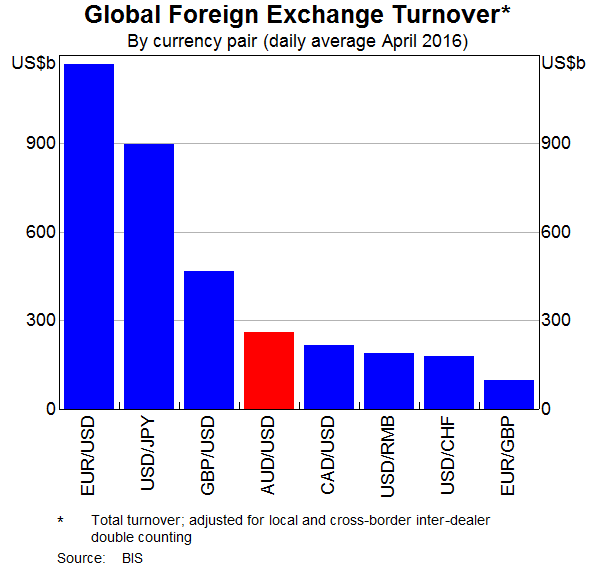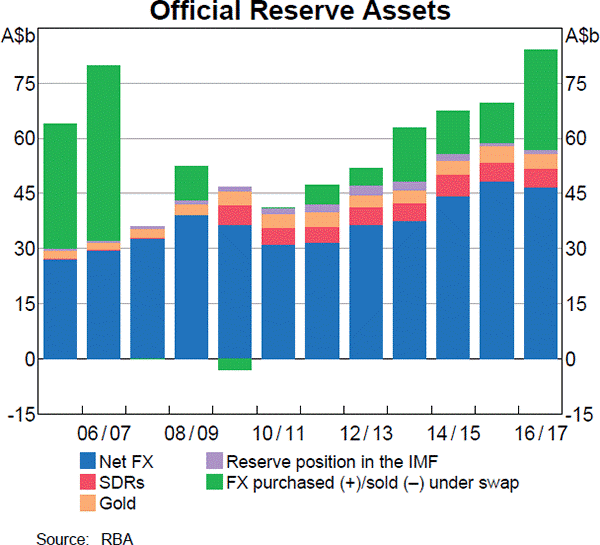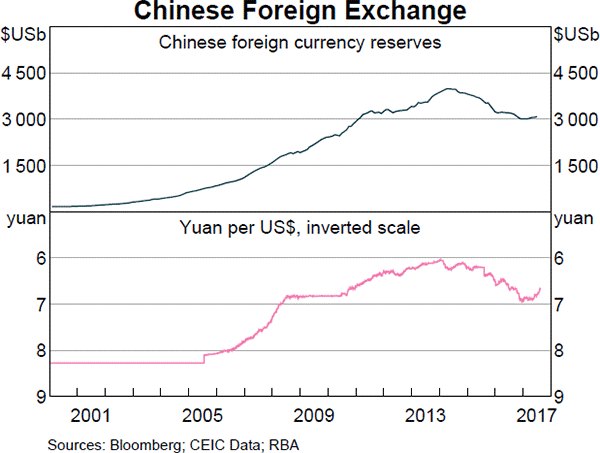# Fight Finance

#### CoursesTagsRandomAllRecentScores

A firm can issue 3 year annual coupon bonds at a yield of 10% pa and a coupon rate of 8% pa.

The beta of its levered equity is 2. The market's expected return is 10% pa and 3 year government bonds yield 6% pa with a coupon rate of 4% pa.

The market value of equity is $1 million and the market value of debt is$1 million. The corporate tax rate is 30%.

What is the firm's after-tax WACC? Assume a classical tax system.

Find Trademark Corporation's Cash Flow From Assets (CFFA), also known as Free Cash Flow to the Firm (FCFF), over the year ending 30th June 2013.

 Trademark Corp Income Statement for year ending 30th June 2013 $m Sales 100 COGS 25 Operating expense 5 Depreciation 20 Interest expense 20 Income before tax 30 Tax at 30% 9 Net income 21  Trademark Corp Balance Sheet as at 30th June 2013 2012$m $m Assets Current assets 120 80 PPE Cost 150 140 Accumul. depr. 60 40 Carrying amount 90 100 Total assets 210 180 Liabilities Current liabilities 75 65 Non-current liabilities 75 55 Owners' equity Retained earnings 10 10 Contributed equity 50 50 Total L and OE 210 180 Note: all figures are given in millions of dollars ($m).

For a bond that pays fixed semi-annual coupons, how is the annual coupon rate defined, and how is the bond's annual income yield from time 0 to 1 defined mathematically?

Let: $P_0$ be the bond price now,

$F_T$ be the bond's face value,

$T$ be the bond's maturity in years,

$r_\text{total}$ be the bond's total yield,

$r_\text{income}$ be the bond's income yield,

$r_\text{capital}$ be the bond's capital yield, and

$C_t$ be the bond's coupon at time t in years. So $C_{0.5}$ is the coupon in 6 months, $C_1$ is the coupon in 1 year, and so on.

Which of the following statements about short-selling is NOT true?

One formula for calculating a levered firm's free cash flow (FFCF, or CFFA) is to use net operating profit after tax (NOPAT).

\begin{aligned} FFCF &= NOPAT + Depr - CapEx -\Delta NWC \\ &= (Rev - COGS - Depr - FC)(1-t_c) + Depr - CapEx -\Delta NWC \\ \end{aligned} \\
Does this annual FFCF or the annual interest tax shield?

One and a half years ago Frank bought a house for $600,000. Now it's worth only$500,000, based on recent similar sales in the area.

The expected total return on Frank's residential property is 7% pa.

He rents his house out for $1,600 per month, paid in advance. Every 12 months he plans to increase the rental payments. The present value of 12 months of rental payments is$18,617.27.

The future value of 12 months of rental payments one year in the future is $19,920.48. What is the expected annual rental yield of the property? Ignore the costs of renting such as maintenance, real estate agent fees and so on. Five years ago you entered into a fully amortising home loan with a principal of$500,000, an interest rate of 4.5% pa compounding monthly with a term of 25 years.

Then interest rates suddenly fall to 3% pa (t=0), but you continue to pay the same monthly home loan payments as you did before. How long will it now take to pay off your home loan? Measure the time taken to pay off the home loan from the current time which is 5 years after the home loan was first entered into.

Assume that the lower interest rate was given to you immediately after the loan repayment at the end of year 5, which was the 60th payment since the loan was granted. Also assume that rates were and are expected to remain constant.

What derivative position are you exposed to if you have the obligation to sell the underlying asset at maturity, so you will definitely be forced to sell the underlying asset?

If the Australian dollar quote of 0.8 USD per AUD suddenly falls to 0.7 USD per AUD, has the Australian dollar or against the US dollar?

Examine the below graphs. The first graph shows daily FX turnover in the world by both the public (government) and private sectors. The second graph 'Official Reserve Assets' shows the FX reserves of the Australian central bank, the RBA. The third graph's top panel shows the FX reserves of the Chinese central bank, the PBoC.Assume that the AUD and USD are priced at parity so 1 AUD = 1 USD.

Which of the following statements is NOT correct?